### Home > CAAC > Chapter 13 > Lesson 13.RE1-S > Problem2-122

2-122.
1. For each of the following expressions, find at least three equivalent expressions. Which do you consider to be the simplest? Homework Help ✎

1. (2x − 3)2 + 5

2.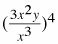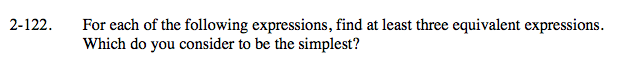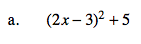Rewrite showing (2x − 3)2 as a product.

(2x − 3)(2x − 3) + 5

Multiply using a generic rectangle.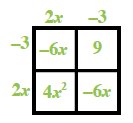Simplify the expression.

(4x2 + (−6x) + (−6x) + 9) + 5
(4x2 + (−12x) + 9) + 5

Simplest Expression: 4x2 − 12x + 14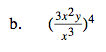Start by writing the expression in the long form.

$\left(\frac{3\textit{x}^{2}\textit{y}}{\textit{x}^{3}}\right)\left(\frac{3\textit{x}^{2}\textit{y}}{\textit{x}^{3}}\right)\left(\frac{3\textit{x}^{2}\textit{y}}{\textit{x}^{3}}\right)\left(\frac{3\textit{x}^{2}\textit{y}}{\textit{x}^{3}}\right)$

Regroup using the Associative and Commutative properties of multiplication.

$\frac{3 \cdot 3 \cdot 3 \cdot 3 \cdot x^2 \cdot x^2\cdot x^2\cdot x^2 \cdot y \cdot y \cdot y \cdot y }{x^3 \cdot x^3 \cdot x^3 \cdot x^3 }$

$\frac{81\textit{y}^{4}}{\textit{x}^4}$